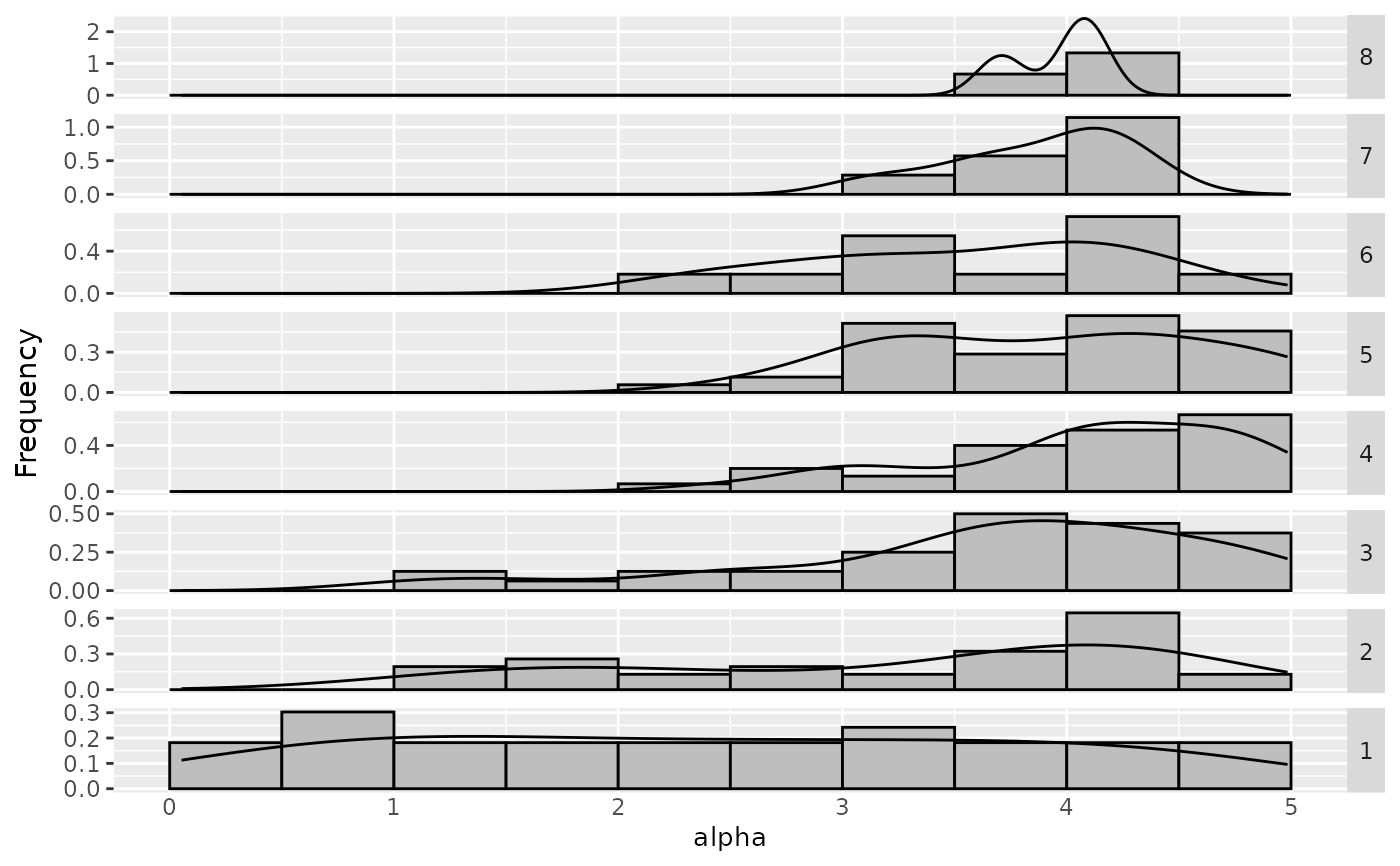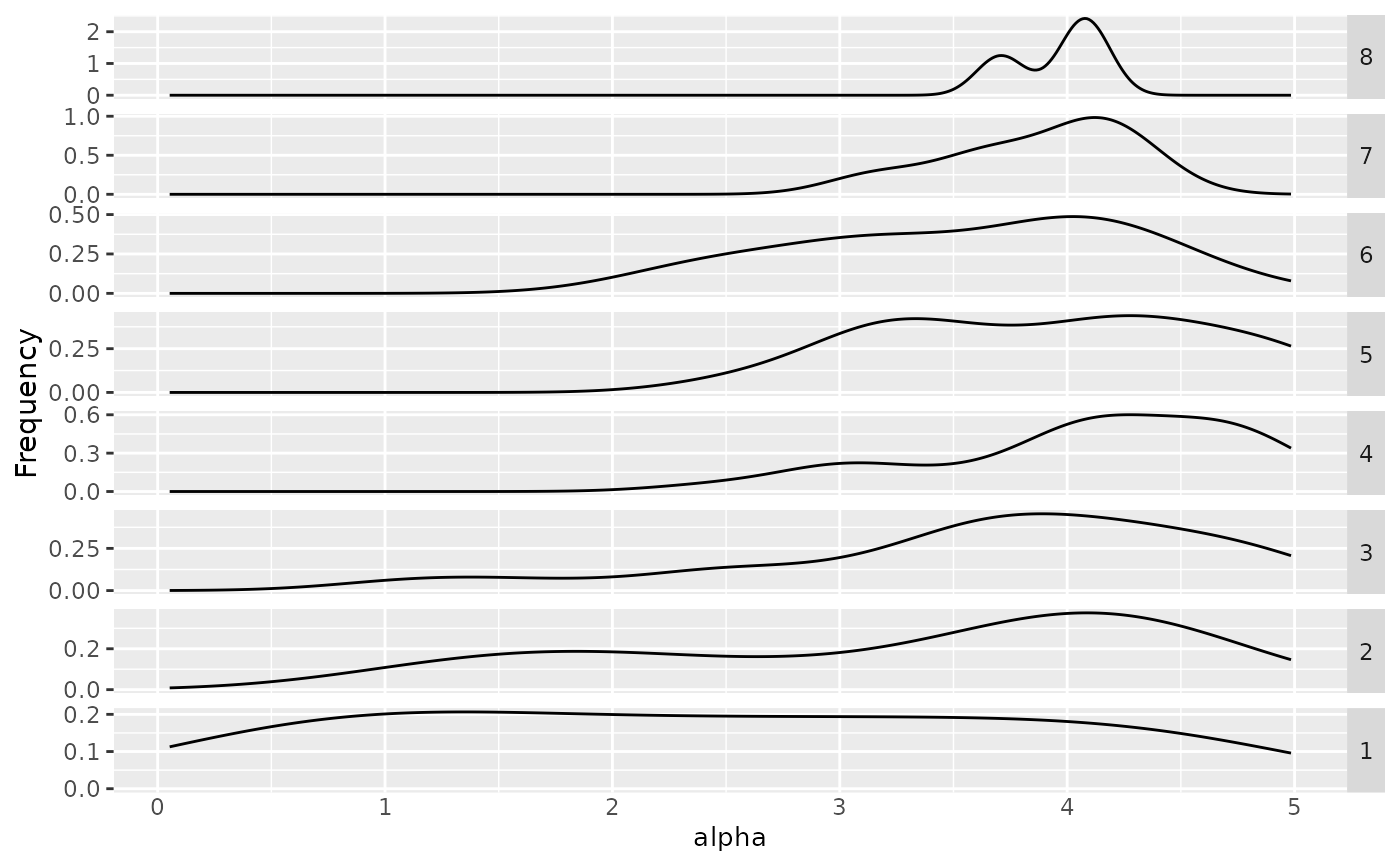The function will return a frequency plot used for categorical data (its values are string, show a bar plot) or numeric data (show a histogram and density plot) by each iteration

sampling_frequency_iteration(
irace_results,
param_name,
numerical_type = "both",
filename = NULL
)

## Arguments

irace_results

The data generated when loading the .Rdata file created by irace (or the filename of that file).

param_name

String, name of the parameter to be included (example: param_name = "algorithm")

numerical_type

String, (default "both") Indicates the type of plot to be displayed for numerical parameters. "density" shows a density plot, "frequency" shows a frequency plot and "both" show both frequency and density.

filename

(character(1)) File name to save the plot, for example "~/path/example/filename.png".

## Value

Frequency and/or density plot

## Examples

iraceResults <- read_logfile(system.file(package="irace", "exdata",
"irace-acotsp.Rdata", mustWork = TRUE))
sampling_frequency_iteration(iraceResults, param_name = "alpha")# \donttest{
sampling_frequency_iteration(iraceResults, param_name = "alpha", numerical_type="density")# }